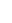Probability-based progressive collapse-resistant assessment for reinforced concrete frame structures Yi Li 1, Xinzheng Lu 2*, Hong Guan 3, Peiqi Ren 2, Lanping Qian 1 1. Key Laboratory of Urban Security and Disaster Engineering of Ministry of Education, Beijing Collaborative Innovation Center for Metropolitan Transportation, Beijing University of Technology, Beijing 100124, China 2. Key Laboratory of Civil Engineering Safety and Durability of Ministry of Education, Tsinghua University, Beijing 100084, China 3. Griffith School of Engineering, Griffith University Gold Coast Campus, Queensland 4222, Australia. Advances in Structural Engineering, Accepted on Mar. 18, 2016. DOI: 10.1177/1369433216649385. Download Full Text Abstract: Progressive collapse is a form of disproportionate damage, which is induced by an initial local failure that propagates throughout a large portion or the entirety of structural systems. The initial local failure may occur anywhere in a structural system, which consequently exhibits different collapse behaviors. However, the progressive collapse resistance is an inherent property of the entire structural system regardless of any specific initial local failure. This paper proposes a collapse probability index to assess the progressive collapse resistance of reinforced concrete (RC) frame structures. Such an index is capable of measuring all typical initial local failures instead of any specific ones. The collapse fragility curve is further established based on the collapse probability index, which varies with an increase in the nominal gravity. The structural redundant resistance to progressive collapse can be assessed by the fragility curve. Furthermore, a simplified assessment method is proposed to measure the collapse probability indices feasibly of RC frames under different nominal gravity levels. The proposed method based on the collapse probability index can comprehensively evaluate the progressive collapse resistance of RC frames which is validated through analysis of a single four-story and a group of eight-story RC frame structures. Keywords: progressive collapse; resistance assessment; reinforced concrete frame structures; collapse probability index; collapse fragility curve; simplified method DOI: 10.1177/1369433216649385 If you need the PDF version of this paper, please email to luxinzheng@sina.com

Introduction

Progressive collapse is a form of damage that is induced by an initial local failure propagating throughout the structural system and eventually resulting in the collapse damage of a large portion or the entirety of the system (Ellingwood, 2006). An initial local failure may be induced by, for instance, a fire, an explosion, an impact, or a human error. Thus, different from the structural performance in resisting various hazards acting on the overall structural system, such as an earthquake or a windstorm, the progressive collapse resistance is the ability of the overall structural system to withstand local destruction. Correspondingly, the progressive collapse-resistant assessment method is also different from the traditional structural assessment methods specific to earthquake or windstorm resistance.

During the last decade, research efforts have been focused on the collapse of local structural elements (Elkoly and El-Ariss, 2014) and the aftermath disproportional collapse of overall structural systems (Murray and Sasani, 2013). The alternate load path (AP) method (Fu, 2009; Kwasniewski, 2010; Bao and Kunnath, 2010; Li and El-Tawil, 2014) or the pushdown method (Khandelwal and El-Tawil, 2008; Kim et al., 2009; Sasani et al., 2011; Li et al., 2016) can intuitively evaluate the collapse mechanism and collapse resistance via the analysis of structural responses, i.e., the force and deformation after the local failure. Further, the relationship between the load to induce collapse and the structural resistance can also be investigated by analyzing the structural energy dissipation and the work done by the gravity load during a collapse process (Izzuddin et al., 2008; Xu and Ellingwood, 2011; Szyniszewskia and Krauthammer, 2012). Such methods can qualitatively assess the progressive collapse performances of various structural systems.

In addition to the qualitative analysis, the quantitative evaluation of progressive collapse resistance is also required in engineering practice to perform tasks such as optimization or strengthening of structures. Hence, many quantitative indices have been proposed to meet such requirement, and these indices can be classified into three levels (Sørensen, 2011): (1) a deterministic index; (2) a probabilistic index; and (3) a risk-based index.

The deterministic index measures the structural collapse resistance through a comparison of the geometric or mechanical performance indices of the overall structural system before and after the local component failure. These performance indices include structural strength (International Standard Organization (ISO), 2007; Bao et al., 2014; Fascetti et al., 2015), failure probability (Lind, 1995), connectivity of the structural form (Agarwal et al., 2003), damage degree (Starossek and Haberland, 2011), stiffness (Starossek and Haberland, 2011; Nafday, 2008), energy dissipation capacity (Starossek and Haberland, 2011; Fang and Li, 2007), natural frequency (Hu, 2007), strain energy (Zhang and Liu, 2007) and so on. The advantage of these indices lies in the clarity of their physical significance and convenience in calculation. On the other hand, although these indices are related to the structural mechanical properties, they do not directly reflect the collapse resistance or the collapse consequence after the failure of a local component. In particular, for the overall structural system, if the initial failure occurs at different locations, the alternate load paths in the system are also different in resisting progressive collapse. Thus, the deterministic index essentially assesses the progressive collapse resistance of given alternate load paths instead of the entire structural system. For the same structural system, different values of the deterministic index are obtained if the initial local failure occurs at different locations, thereby resulting in significantly diverse assessment outcomes. In reality, however, the progressive collapse resistance should be independent of a specific initial failure because it is an inherent natural characteristic of an overall structural system.

The probabilistic index can be described by the following equation (Ellingwood and Dusenberry, 2005):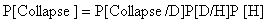(1)

where P[Collapse] and P[H] are the probability of a structural collapse and that of an accident, respectively; P[D/H] is the conditional probability of the initial local failure induced by an accident; and P[Collapse/D] is the conditional probability of the progressive collapse induced by a local failure. The risk-based index is established based on the probabilistic index (Equation (1)), with further consideration of the consequences caused by a progressive collapse, such as economic losses and casualties (Sørensen, 2011). This index can provide a more comprehensive assessment of the collapse accident, which offers useful assistance to design decisions (Sørensen, 2011; Baker et al., 2008). Evidently, both the probabilistic index and the risk-based index rely on the probabilities P[H], P[D/H] and P[Collapse/D]. If all the possible accidents and initial local failures can be rationally considered when calculating these three probabilities, then the resulting assessment index is capable of fully reflecting the progressive collapse resistance of a structure. However, it is difficult to accurately determine these three probabilities, especially when there is a lack of the related statistical information of the building site (Starossek and Haberland, 2011).

A collapse probability index, i.e., a probabilistic index, is proposed in this work through an AP analysis examining all the initial local failure scenarios suggested by current design codes. Consequently, such an index is independent of any specific local failure scenario and can therefore objectively assess the progressive collapse resistance of an overall structure. A progressive collapse fragility curve is also established based on the collapse probabilities under different nominal gravity levels by which the redundant collapse resistance of structures can be described. A simplified assessment method to obtain the collapse fragility curve is further proposed, with which the computational workload is substantially reduced to facilitate engineering practice. Finally, this assessment method is applied to evaluate the progressive collapse performance of a single four-story and a group of eight-story reinforced concrete (RC) frame structures.

The collapse probability index

The progressive collapse resistance is the ability of the overall structure to resist local failure. In Equation (1), the probability of an accident P[H] and the conditional probability of the initial local failure P[D/H] are independent of the structural collapse resistance (Starossek and Haberland, 2011). Thus, it can be assumed that P[D/H]P[H]=1 and that only the conditional probability of the progressive collapse P[Collapse/D], namely the collapse probability after the initial failure, is used to evaluate the structural progressive collapse performance.

In a study of earthquake-induced collapse, Zareian and Krawinkler (2007) proposed a collapse probability to evaluate the vulnerability of structures under seismic action. In their study, a total of 40 seismic ground motions were chosen as the standard input, and the collapse probability was defined as the ratio of the number of ground motions that would induce collapse to the total 40 ground motions. When representative ground motions are selected, for example, covering different frequency spectra and durations, the collapse probability of the structures subjected to these seismic actions can then reflect the differences of the seismic resistance of these structures. During progressive collapse analysis and design of building structures (General Services Administration (GSA), 2003; Department of Defense (DoD), 2010), representative vertical support components on different floors are removed to investigate the structural collapse response due to the local failure. Similar to the earthquake-induced collapse probability, when the number of removal scenarios is sufficient and the removed structural members are representative, the progressive collapse resistance of the structural system can then be reflected by the ratio of the number of collapse scenarios to the total number of removal scenarios. Therefore, the progressive collapse probability, i.e., P[Collapse/D], can be expressed as: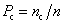(2)

where nc and n are the number of the collapse scenarios and that of all the removal scenarios, respectively. Note that in the analysis, considering the characteristics of loading and structural symmetry, only the representative components must be removed; this in turn significantly reduces the computational workload. For the RC frames with regular structural configuration, the typical columns (e.g., a corner column, two edge column and an interior column) can be selected as the representative components, according to the GSA and DoD guidelines (GSA, 2003; DoD, 2010). For the RC frames with irregular structural configuration, in addition to the typical columns, more columns should also be chosen as the representative components based on a thorough progressive collapse risk analyses, following the corresponding specifications such as the European Committee for Standardization (ECS, 2006). For example, the key columns that are unlikely to be bridged over by the residual structural system should be identified as the representative components through the alternate load path analysis. Further, the columns that are vulnerable to disastrous actions should also be identified through the risk analysis. The collapse criterion regulated by the DoD guideline (2010) is adopted in this study, in which the entire structures are considered to have collapsed when the remaining structures (i.e., the initially non-damaged portion) exceed the allowable structural deformation. For the RC frame structures investigated in this study, the allowable structural deformation refers to the rotation of beams and columns not exceeding 0.2 rad (DoD, 2010).

Progressive collapse fragility curve

Using the method described above (Equation (2)), the structural collapse probability, namely, how likely collapse will be triggered in a structure under the action of the design gravity load, can be obtained. Note that, due to the random nature of the load acting on the structure, the collapse probability under the design gravity load cannot comprehensively assess the progressive collapse resistance of building structures. To address this issue, the redundant structural collapse resistance is further considered in research, engineering design and optimization. In earthquake engineering, the collapse fragility curve is widely used to evaluate the safety margins against earthquake-induced collapse of structural systems (Applied Technology Council (ATC), 2009). The incremental dynamic analysis (IDA) (Vamvatsikos and Cornell, 2002) is used to obtain the fragility curves. In other words, the structural collapse probabilities under different intensities of earthquakes are obtained by gradually increasing the earthquake action to the structure. Consequently, referring to the concept of IDA, the progressive collapse fragility curve of building structures can also be established to evaluate the structural safety margins against progressive collapse (i.e., the redundant resistance). Specifically, during the progressive collapse analysis, by gradually changing the magnitudes of the external load (i.e., the gravity load) acting on the structural system, the corresponding collapse probabilities under different loading levels can then be calculated to establish the progressive collapse fragility curve.

An example of the progressive collapse fragility curve is illustrated in Figure 1, where the vertical axis is the collapse probability index presented in Equation (2), and the horizontal axis is the selected intensity measure (IM), namely, the nominal gravity. When IM = 1.0G (G is the gravity load), the corresponding collapse probability is the collapse probability subjected to the design load. For a structure with insufficient collapse resistance, the insufficiency can be represented by the part of the curve where IM is less than 1.0G. Similarly, the redundant resistance of the structural system can be represented by the part of the curve where IM is greater than 1.0G. For a structure that is designed to prevent progressive collapse and completely meet the collapse resistance demand, the collapse probability should be zero under any expected initial local failure, (i.e., Pc = 0 when IM = 1.0G), which is shown in Figure 1 as the ��Robust structure��. In this case, the entire fragility curve describes the progressive collapse resistance margin of the structure.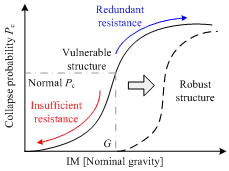Fig. 1  Progressive collapse fragility curve

Numerical examples of proposed probability index and fragility curve

An 8-story RC frame structure (Model A) is analyzed to illustrate the proposed collapse probability index and the progressive collapse fragility curve. The perspective and plan view of Model A are shown in Figure 2. According to the regulations specified in the GSA (2003) and DoD (2010) guidelines, the representative removed columns (i.e., a corner column, two middle edge columns and an interior column) on each story are selected (see Figure 2b). Each removal column represents a number of the columns at the similar location of the structural plan. The number of these represented columns is considered in Equation (2) when calculating the collapse probability index. The height of the first story is 4.2 m, and that of the remaining stories is 3.6 m. The structure is fully fixed to the ground at the first floor. Tables 1 and 2 list the structural member sizes, material strengths and the loading information. Note that these tables also include additional information for subsequent analyses presented in Section 6. Model A is designed according to the Chinese Code for the Design of Concrete Structures (GB50010-2010) (The Ministry of Housing and Urban-Rural Development of the People��s Republic of China (MOHURD), 2010a) and the Code for Seismic Design of Buildings (GB50011-2010) (MOHURD, 2010b).

Table 1.  Structural parameters of the RC frames

 Beam Column Slab Sections 8-story model Longitudinal beams (X-direction): 300 �� 500 mm Transverse beams (Y-direction): 300 �� 550 mm 550�� 550 mm 120 mm (thickness) 4-story model Peripheral beams: 300 �� 500 mm Interior beams: 250 �� 450 mm 500�� 500 mm 120 mm (thickness) Concrete C30 ( compressive strength 20.1 MPa) Reinforcing steel Longitudinal reinforcement: HRB335 (yield strength 335 MPa)   Hoop reinforcement: HPB235 (yield strength 235 MPa)

Table 2.  Design loads and seismic action on the RC frames

 Seismic action* 8-story model Model A: 200 cm/s2, Model B: 100 cm/s2, Model C: 50 cm/s2 4-story model Model D: 200 cm/s2 Dead load Floor: 5.0 kN/m2, Roof: 7.5 kN/m2 Live load Floor: 2.0 kN/m2, Roof: 0.5 kN/m2 Wind Load Wind pressure: W0��0. 45 kN/m2 Gravity load of a filled wall Floor: 8.0 kN/m, Roof: 6.0 kN/m

*PGA (peak ground acceleration) of the design earthquake (i.e., a 10% probability of exceedance in 50 years)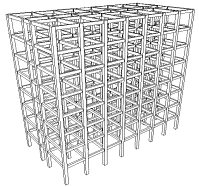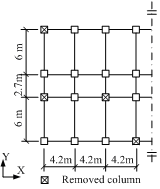(a)  Perspective view (b)  Plan view Fig. 2  An 8-story RC frame

The numerical model of the 8-story RC frame is built using the fiber element model THUFIBER developed at Tsinghua University (Lu et al., 2013), as shown in Figure 3a. In this model, the cross sections are divided into a number of individual fibers which satisfy the plane section assumption. Uniaxial constitutive laws for steel and concrete are assigned to different fibers. This model can accurately simulate the nonlinear behavior of the RC beam or column members under large deformations, for which the dominant failure is flexural or tensile failure. Details of the model including the basic assumptions, elemental failure criteria and modeling techniques can be found in the published literature (Lu et al., 2013). This model has also been validated by the typical progressive collapse tests of RC frame structures (Ren et al., 2014; Sadek et al., 2011; Yi et al., 2008), as shown in Figure 4, where L is the clear span of the tested frames and Pbmax is the peak load of the frames under the beam mechanism. It can be seen that both the beam action and the catenary action developed in the RC frames can be accurately simulated. This model has also been successfully applied in the progressive collapse analyses of building structures (Li et al., 2011, 2014a, 2014b; Ren et al., 2015). In the present study, this element model is used to simulate the beams and columns of the RC frames, as shown in Figure 3b.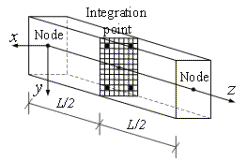(a) Fiber beam element (b) Element mesh Fig. 3  Numerical model of the example structures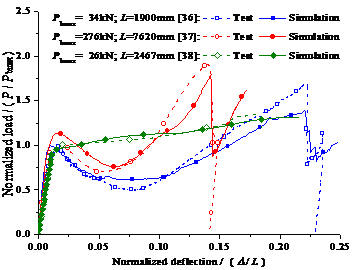Fig. 4  Validation of the fiber beam model to simulate the progressive collapse tests

An accurate nonlinear dynamic AP (NDAP) analysis is conducted to obtain the structural collapse probabilities subjected to different normalized vertical loads, which are marked as squares in Figure 5 under 32 nominal gravity levels. Eads et al. (2013) proved that structural collapse probabilities under earthquake action follow a lognormal distribution. To better explain the variation of the progressive collapse probability subjected to the nominal gravity, the lognormal distribution function is also used herein to fit the progressive collapse fragility curve, as shown in Figure 5. The fitted curve passes the Kolmogorov�CSmirnov test at a 5% significance level, confirming that the structural collapse probability under the progressive collapse condition also follows a lognormal distribution (Benjamin and Cornell, 1970). In view of this, the lognormal distribution is used to fit the progressive collapse fragility curves thereafter.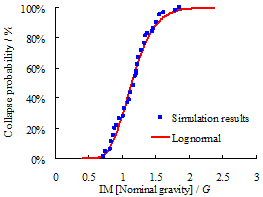Fig. 5  Progressive collapse fragility curve of Model A

The simplified assessment method

Following the NDAP analysis, the collapse probability index is calculated to determine whether collapse will occur after the initial failure of local components (GSA, 2003; DoD, 2010). It should be noted that the nonlinear dynamic analysis is very time-consuming. In particular, when establishing the progressive collapse fragility curve, the structural dynamic responses to the initial failure must be calculated at various locations and under different nominal gravity loads, which results in an excessively high computation workload. For the case shown in Figure 5, a total of 1024 nonlinear dynamic analyses are required to obtain the fragility curve. Thus, a simplified assessment method based on the nonlinear static pushdown analysis is proposed in this section to accelerate the calculation process of the collapse fragility curve of RC frames.

In the nonlinear static pushdown analysis, a gradually increased load, i.e., a pushdown load (see Figure 6a) is applied to the collapse-resisting substructure, in which the critical vertical component is removed, to obtain the structural resistance-deformation curve (Khandelwal and El-Tawil, 2008) (Figure 6b). Note that the gravity load acting on the remaining structure remains unchanged. The maximum load on the curve within the allowable structural deformation refers to as the maximum collapse resistance Rmax of the substructure after the component fails. For a RC frame structure shown in Figure 6a, the maximum collapse resistance Rmax may be achieved under the beam mechanism or the catenary mechanism (Li et al., 2011, 2014a, 2014b), represented by the two types of structural resistance curves as indicated in Figure 6b.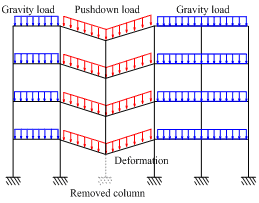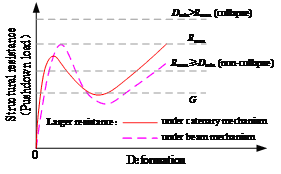(a) Load pattern (b) Structural resistance curve Fig. 6  Collapse resistance assessment via pushdown analysis

Progressive collapse is induced by the unbalanced gravity load G (which is supported by the initial failed component) acting on the remaining collapse-resisting substructure. Note that most initial damages caused by an accident are instantaneous (e.g., explosion and collision), therefore dynamic effects do exist in the collapse process which amplify the internal forces in the structure. This results in Dmin�RG, in which Dmin is the minimum demand of the structure to effectively resist progressive collapse. If the maximum resistance Rmax is larger than or equal to the minimum resistance demand Dmin, then the structure will not collapse. Otherwise, if Rmax is less than Dmin, the structure will collapse, as is shown in Figure 6b.

The structural minimum resistance demand Dmin can be approximately calculated by the following equation:(3)

where DAF is the dynamic amplification factor which is also named as the dynamic increase factor (DIF) in the DoD guideline (2010). Note that DAFs for the demands under the beam mechanism and the catenary mechanism are distinctly different. Hence, the value of DAF should be assessed according to the types of the structural resistance curves (the maximum resistance under the beam mechanism or the catenary mechanism as shown in Figure 6b). GSA (2003), DoD (2010) and Li et al. (2014b) provide the DAF values for RC frame and shear wall structures under the beam mechanism, and Li et al. (2014a) suggested a DAF value for RC frame structures under the catenary mechanism. These proposed DAF values can be utilized to evaluate the structural minimum resistance demand Dmin which in turn assess if progressive collapse would occur.

Note that Rmax can be obtained by the nonlinear static pushdown analysis. The consequence of initial local failure can be evaluated by comparing the values of Rmax and Dmin. Based on the above analysis, the simplified progressive collapse resistance assessment method is proposed:

(1)                Establish a numerical model of the overall structure and conduct a nonlinear static pushdown analysis to obtain the maximum resistance Rmax under a representative removal scenario.

(2)                Calculate the DAF value based on the existing formulas (GSA, 2003; DoD, 2010; Li et al., 2014a, 2014b), and substitute the nominal gravity into Equation (3) to calculate the structural minimum resistance demand Dmin. Compare Rmax and Dmin to determine whether progressive collapse will occur under this removal scenario.

(3)                Repeat Step (1) and Step (2) for all representative removal scenarios under the nominal gravity. Based on all the analysis results, calculate the collapse probability index Pc of the overall structure according to Equation (2).

(4)                Continuously increase the nominal gravity and repeat Step (1) to Step (3) to obtain different values of the structural collapse probability index Pc under a series of nominal gravity levels. Plot the progressive collapse fragility curve, as illustrated in Figure 1, and then conduct the progressive collapse resistance assessment.

The proposed method can significantly reduce the computational effort because: (1) the calculation workload of the nonlinear pushdown analysis is significantly less than that of an equivalent nonlinear dynamic analysis; and (2) for each representative removal scenario, only a single nonlinear static pushdown analysis is required to be conducted. In addition, after the value of Rmax is obtained, the collapse probabilities under different nominal gravity levels can be evaluated. Thus, the calculation workload is significantly reduced when compared with the NDAP analysis, in which the nonlinear time-history analysis is required for each representative removal scenario under each nominal gravity scenario.

Using the proposed simplified assessment method, Model A is re-analyzed. The value of DAF is essentially important for the accuracy and efficiency of calculating the progressive collapse demand. GSA (2003) and DoD (2010) are two mostly influential design guidelines and engineers are well familiarized with their design procedures. Note that the DAF specified in the GSA guideline is simpler for engineering application due to its independence of the structural ductility as compared to that in the DoD (2010). Therefore, the DAFs proposed in the GSA guideline are selected herein to calculate the structural minimum resistance demand Dmin. Also note that the actual DAF in the GSA guideline is combined by a nominal DAF and a demand capacity ratio (DCR) which considers the dynamic effect and the nonlinear material behavior during the collapse process respectively. Two values of DAF, 1.0 and 1.33, are suggested in the GSA guideline. Extensive existing analysis results show that the DAF of frames is between 1.2 and 1.5 (Ruth et al., 2006; JalaliLarijani et al., 2013). Hence, the value of 1.33 is chose as the DAF of the numerical examples in this study. Although such a fixed DAF value may not be the most accurate one, it is quite simple, widely accepted by engineers, and accurate enough that will be proved in the following analysis.

The lognormal distribution function is also used to fit the progressive collapse fragility curve, as presented in Figure 7. The collapse fragility curve obtained by the simplified method is found to conservatively agree with that obtained by the NDAP method. The average difference of the collapse probabilities is 10.8% between the predictions of the proposed simplified method and those 32 numerical results given in Figure 5. Note that the accuracy of the simplified method is influenced by the DAF value. When the error of the DAF value is acceptable for engineering design, such as those suggested by the DoD and the GSA guidelines, the progressive collapse resistance assessment results are considered acceptable. In addition, if all the structures are evaluated with the same probability index and the same analysis method, the relative value of the assessment results is still convincing. Thus, for highly important structures, the accurate method, i.e., the NDAP method, is recommended to assess the progressive collapse resistance. In the design of normal structures or preliminary analyses of highly important structures, the proposed simplified method is recommended due to its simplicity and convenience.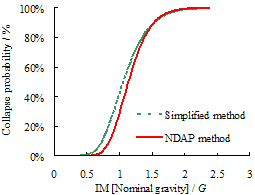Fig. 7  Progressive collapse fragility curves based on the simulation results of different methods (Model A)

Numerical examples using the proposed assessment method

Frame model information

Based on Model A, two new RC frame models, named as Model B and Model C, are generated, with detailed information presented in Table 2. The two models are consistent with Model A in structural layout and dimensions, material strength and site soil conditions. However, two different seismic design intensities (meaning that the values of seismic load are different) are considered in the structural design of Models B and C. Subjected to different design seismic loads, i.e. different progressive collapse resistance, the reinforcement ratios are different in Models A, B and C. For each structure, two numerical models are established: Model X�CS and Model X, with and without considering the slab effect, respectively; where X represents different seismic action, i.e., A, B and C (see Table 2). This results in a total of six models for the 8-story frame structure. The frame beam is modeled by a T-shaped or a L-shaped fiber beam element where its flange represents the slab (Sasani, 2008). The capacity of the fiber beam model to simulate a T-shaped or a L-shaped RC structural element is validated through comparison with experimental tests of RC columns (Shah, 1984; Sun, 2006), as shown in Figures 8 and 9. Note that the catenary action through the beams and their flanges can be considered in this model whereas the tensile membrane action in the slabs is neglected. By comparison of the analytical results of these models, the performance of the proposed collapse probability index and fragility curve under varying structural design parameters can be evaluated, and thus the rationality and reliability of the proposed index and curve can be validated.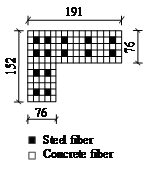(a) Section mesh (units: mm) (b) Comparison of the results Fig. 8  Validation of L-shaped fiber beam model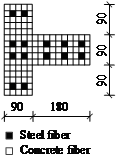(a) Section mesh (units: mm) (b) Comparison of the results Fig. 9  Validation of T-shaped fiber beam model

In addition, a 4-story frame structure (Model D) is also designed in this work. Model D and Model A have the same seismic design intensity (i.e., a design PGA = 0.2 g for 10% probability of exceedance in 50 years) and the same overall floor area (approximately 3600 m2). However, their dimensions, including the number of spans, the span lengths and the sectional sizes of members, are different, as is presented in Table 1 and Figure 10. The other design parameters remain unchanged. In view of the structural layout, Model D is symmetric about both X- and Y-axes, given that the same moderate span is chosen in both directions. Model A, a tall frame with rectangular plan view and Model D, a low-rise frame with symmetrical plan view, are considered two design options for a new building. Having the same overall floor area, both of these options meet the functional requirement. The proposed assessment method is used herein to determine which option produces a higher progressive collapse resistance.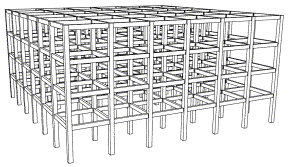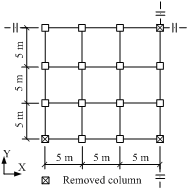(a)  Perspective view (2)  Plan view Fig. 10  4-story RC frame (Model D)

Using the simplified assessment method, all six of the 8-story models are analyzed; their collapse fragility curves are plotted in Figure 11. With an increase in the design seismic intensity, the structural fragility curve is found to shift rightward, which means that the redundant progressive collapse resistance is improved, i.e., the collapse probability of the structural system subjected to the same initial damage is reduced. This outcome is achieved because the seismic design increases the reinforcement ratio and in turn improves the capacity of the structural components, thereby enhancing the progressive collapse resistance. For the frames without considering the slab contributions (Figure 11a), the design PGA is increased from 0.05 g (Model B) to 0.10 g (Model C), while the collapse probability is only decreased by 8% at IM = 1.0G. Whereas, the design PGA is increased from 0.10 g (Model B) to 0.20 g (Model A), with a decrease of 45% in the collapse probability at IM = 1.0G. This is because when the design seismic intensity is relative low (e.g., Models B and C), the amount of reinforcement is primarily governed by the gravity load. Although the seismic action of Model B doubles that of Model C (Table 2), the reinforcement amount in the beams of Model B (17.88 ton) is only slightly higher than that of Model C (14.80 ton). For Model A under a much higher design seismic intensity, its reinforcement amount in the beams (30.8 ton) is much higher. Thus Model A exhibits a significantly improved progressive collapse resistance. A similar phenomenon can also be observed in the frames considering the slab contributions (Figure 11b). The above comparison indicates that the characteristics of the collapse probability and fragility agree entirely with the theoretical analysis. The proposed probability index and fragility curve can well reflect the progressive collapse performance of the overall structure.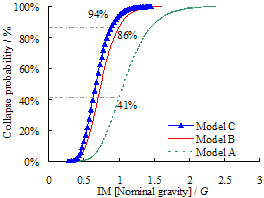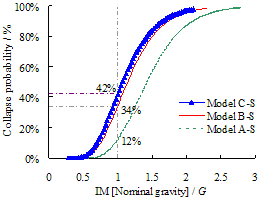(b)  Frames with slab contributions Fig. 11  Progressive collapse fragility curves for frames with different seismic designs

Frame models with or without consideration of slab contributions

Figure 12 shows the progressive collapse fragility curves of Model A and Model C. For each model, the slab effects (Model A-S and Model C-S) are also examined. The collapse fragility curves for Models A-S and C-S are found to significantly shift rightward relative to Models A and C. This outcome suggests that considering the slab contribution results in a much larger progressive collapse resistance. An existing experimental study (Qian and Li, 2012) indicates that the slabs can greatly enhance the progressive collapse resistance of RC frame structures, in which the flexural and axial strengths of the beams can be largely improved by concrete flanges and longitudinal reinforcing bars in the slabs. This comparison on the slab effect again confirms the rationality of the collapse probability index and the fragility curve proposed in this work.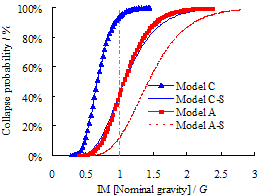Fig. 12  Progressive collapse fragility curves for frames with and without consideration of slab contributions

Frame models with different structural layouts

The progressive collapse fragility curves for Models A and D with different structural layouts are presented in Figure 13. The curve of Model D is found to be significantly shifted to the left compared with that of Model A. This implies that the collapse resistance of Model D is much lower than that of Model A. Consequently, the collapse probability of Model D is significantly higher than that of Model A under the same level of nominal gravity. For example, when IM = 1.0G, the collapse probability of Model D, which equals 81%, is 40% higher than that of Model A.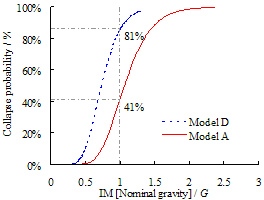Fig. 13  Progressive collapse fragility curves for frames with different layouts

To quantitatively evaluate the progressive collapse resistance of the two structures, the NDAP analysis (GSA, 2003; DoD, 2010) is conducted to improve the progressive collapse resistance capacity of the two models by increasing the reinforcing steel in the beams. For Model A and Model D to achieve the progressive collapse-resistant design target (i.e., the collapse probability equals 0% when IM = 1.0G), the percentage increases in the amount of steel are 2.47% and 11.78%, respectively (see Table 3). It is evident that the design target is much easier to be achieved by Model A than by Model D. Such a difference on the progressive collapse redundancy is also clearly illustrated in the fragility curves (Figure 13).

Table 3. Comparison of steel amount in the beams of Model A and Model D

 Floor Model A Model D Original Collapse prevention design Original Collapse prevention design SA SA Ic SA SA Ic 8 2.00 2.27 13.50% �� �� �� 7 2.33 2.64 13.30% �� �� �� 6 2.91 3.09 6.19% �� �� �� 5 3.64 3.64 0 �� �� �� 4 4.26 4.26 0 4.02 5.60 39.30% 3 4.87 4.87 0 5.19 6.33 21.97% 2 5.36 5.36 0 7.05 7.20 2.13% 1 5.43 5.43 0 8.11 8.11 0 Total 30.80 31.56 2.47% 24.37 27.24 11.78%

Note: SA �C Steel amount in ton; Ic �C Increase as compared to the original structures

It can be further concluded that, for the two structural layouts which have approximately the same overall floor area, the taller structure (Model A) has a higher progressive collapse resistance than the lower one (Model D). This is because both structures are designed using a relatively large design earthquake intensity; therefore reinforcing steels in Model A and Model D are governed by the seismic load. Note that the seismic load of Model A is larger than that of Model D. As such, the structural components of Model A have larger bending and shear capacities than those of Model D, resulting in a larger progressive collapse resistance of Model A. All the above observations again confirm that the outcome of the proposed assessment method agrees with the theoretical analysis. In addition, the fact that Model A is much easier to achieve the progressive collapse-resistant design target than Model D, is a good example to demonstrate that the proposed fragility curve can quantitatively evaluate the progressive collapse redundancy of different structures.